# Representing Half

in Educationlast year

Hi there. In this math education post, I cover the concept of half.

Math text rendered in LaTeX (lay-tech) with QuickLatex.com.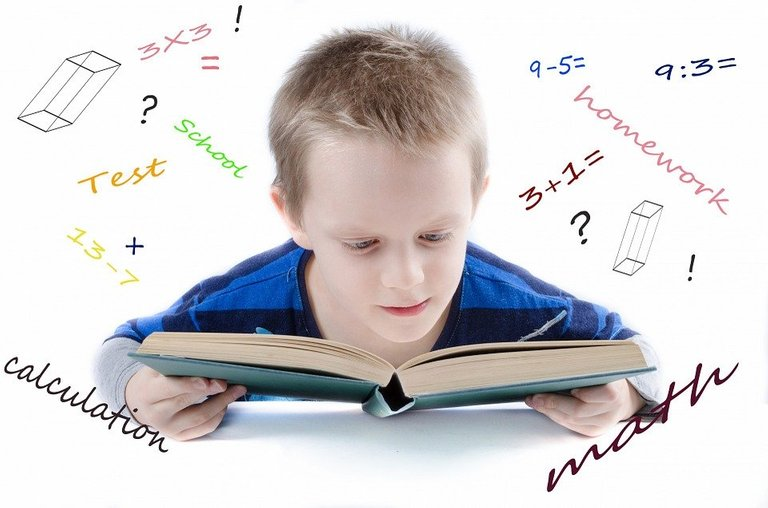Pixabay Image Source

## Topics

• Half As Two Equal Parts Of A Whole
• Taking Half Of Numbers
• Equivalent Fractions To A Half
• Percents To Represent Half
• Decimals To Represent Half

## Half As Two Equal Parts Of A Whole

I think one of the best ways to understand the concept of a half is through a picture. Consider a big round cake. Taking half of a cake or any object involves splitting it into two equal pieces and then take one of the two pieces.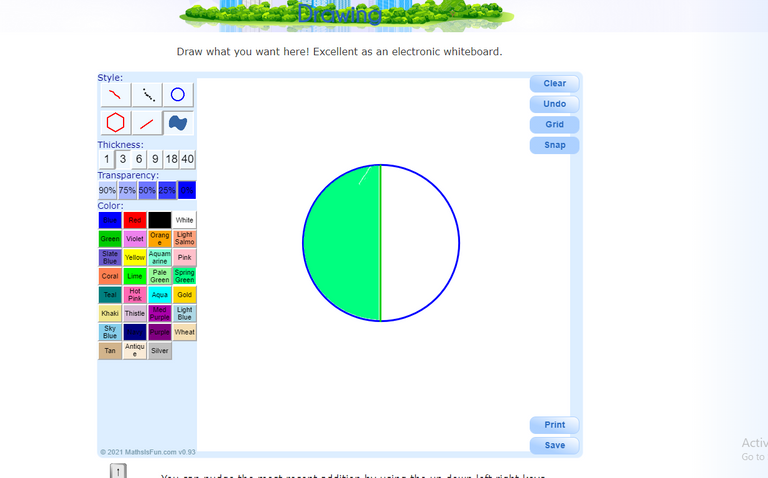Mathisfun Drawing Tool Screenshot

## Taking Half Of Numbers

When it comes to taking half of a number, think of it as something plus itself to get that number. Half of 20 is 10 as 10 plus 10 gives the twenty. Half of eight is four as 4 + 4 is 8.

The examples so far have dealt with taking half of even numbers. Taking half of odd numbers is a bit more tricky. Odd numbers contain one plus an even number. Consider the number five where it is `1 + 4`. Half of four is two and half of one is half. Half of 5 is 2 and a half which can be written as `2 1/2`.

Examples

• Fifty is half of 100.

• Half of 15 is 7 and a half.

• Half of 2000 is 1000.

• 12 and a half is half of 25.

## Equivalent Fractions To A Half

There are multiple ways to represent half in fractions form. The basic representation for half is `1/2`. With the use of equivalent fractions half can be represented in other ways.

Half can be represented as 2 out of 4 or two fourths. This is from cutting a whole cake into 4 equal pieces and taking two of the four.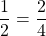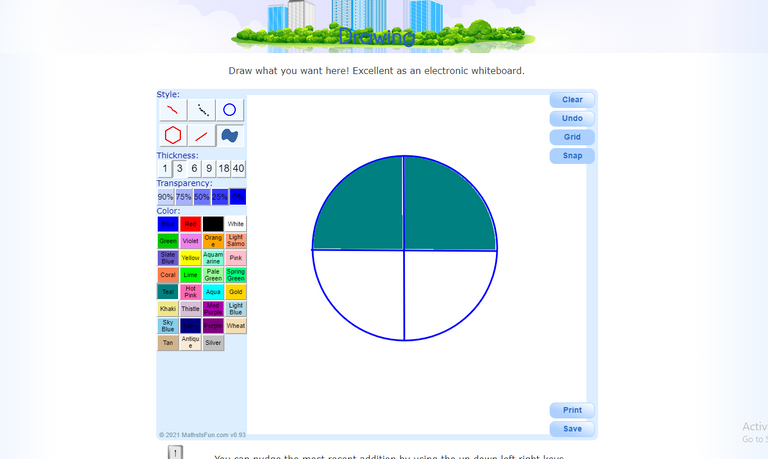Mathisfun Drawing Tool Screenshot 2

Another representation of half is 4 eighths. This is taking 4 from 8 equal pieces of the whole.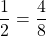Other representations of half includes these fractions.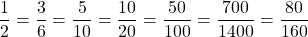## Percents To Represent Half

Fractions can be related to percents. One percent is 1 out of 100 which is 1/100 as a fraction.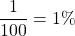Half is one out of two. What is the percentage for half if half is 1 out of 2? With the usage of equivalent fractions, the equivalent fraction for 1 out of 2 is 50 out of 100. Multiply the top and bottom by 50 on one half. This 50 out of 100 is 50% which is for one half.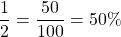## Decimals To Represent Half

The value of a half can be represented as a decimal number. Convert the fraction of a half to an equivalent fraction that has a 10, 100, 1000, etc. in its denominator. Note that one tenth is 0.1 which is from 1/10. One hundredth is 1/100 which has a decimal form of 0.01.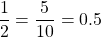Expressing half as 0.5 is a common and simple decimal form. You could add more zeroes behind the five but it is not needed. Here is how 0.50 is converted from 1/2.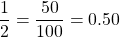You could also express half as 0.500.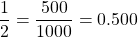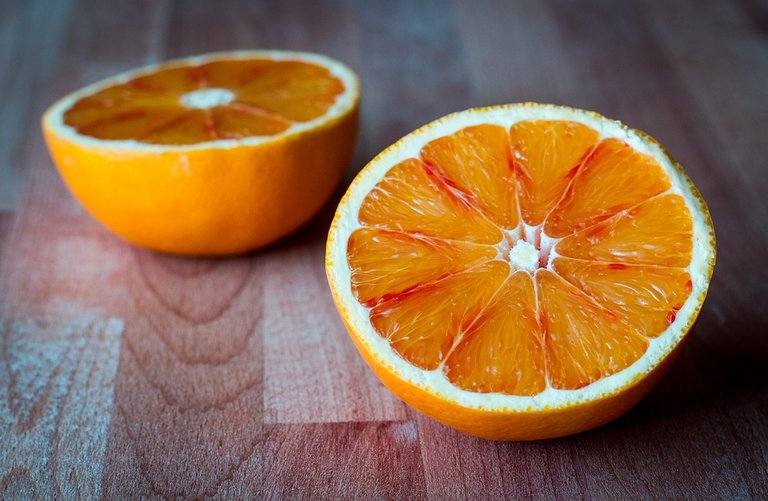Pixabay Image Source